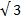# If  |A×B| =(A.B) , then what is the value of |A+B|.

5 years ago
Dear Ravi

|A×B|/A.B=√3

=> ABsin x / ABcos x= √3 (Considering the angle between the two vectors as x)

=> tanx =√3

=> x= π/3

=> |A+B|=√(A^2 + B^2 +2AB cos x)

=> |A+B|=√(A^2 + B^2 +2AB* 1/2) [Since cos π÷3 = 1/2]

=> |A+B|=√(A^2 + B^2 + AB)

Regards